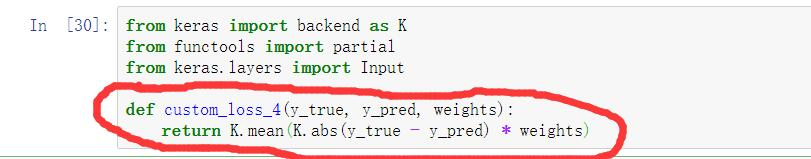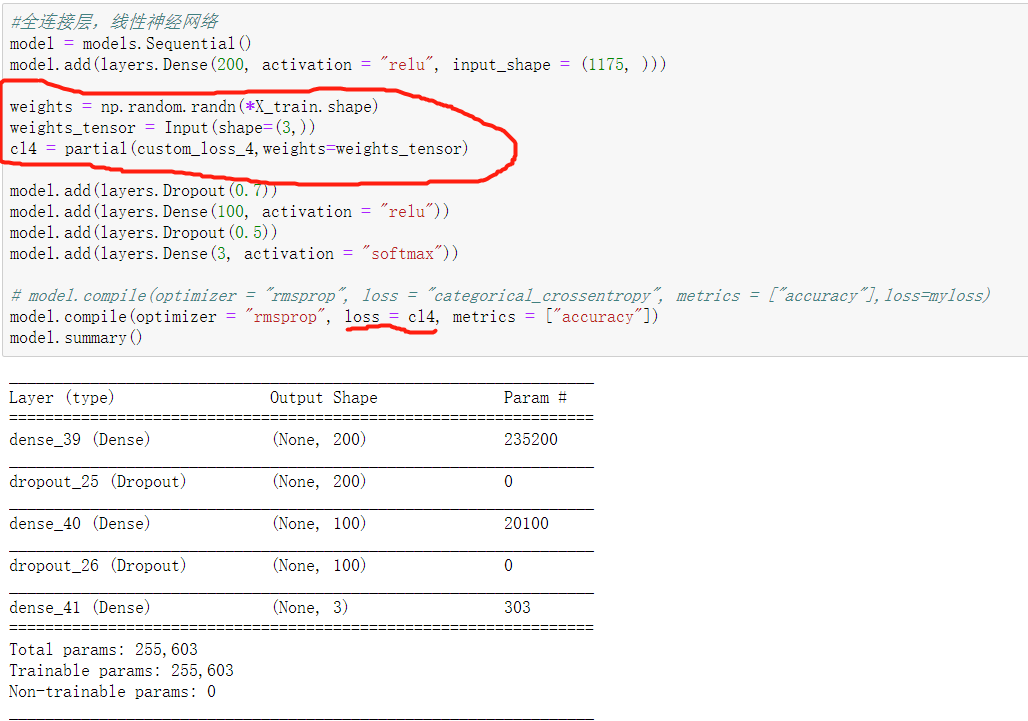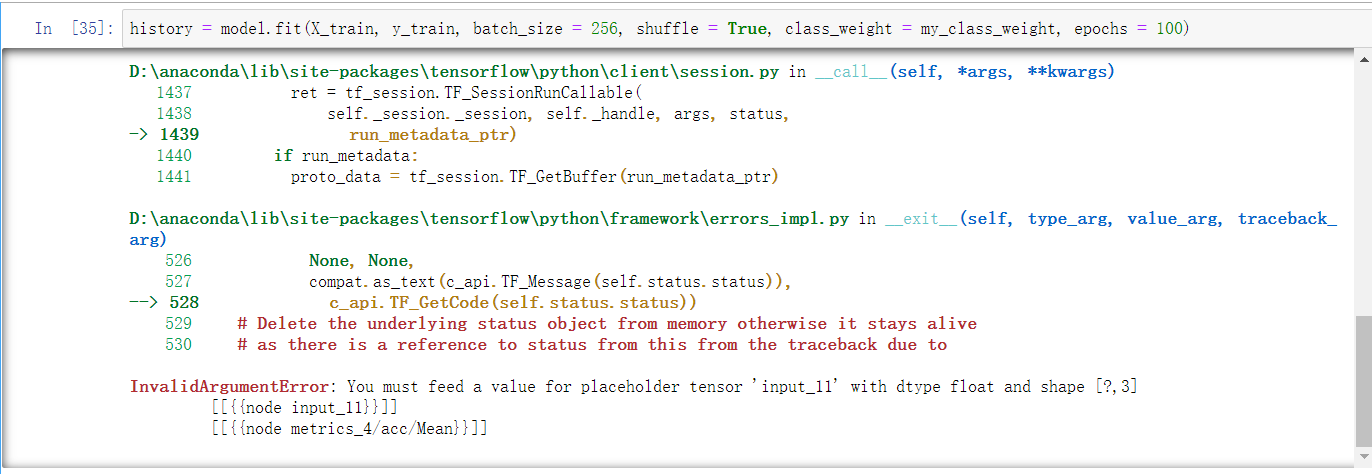python如何自定义权重损失函数？ 80C

def custom_loss_4(y_true, y_pred, weights):
return K.mean(K.abs(y_true - y_pred) * weights)
model = models.Sequential()
model.add(layers.Dense(200, activation = "relu", input_shape = (1175, )))
weights = np.random.randn(*X_train.shape)
weights_tensor = Input(shape=(3,))
cl4 = partial(custom_loss_4,weights=weights_tensor)
model.compile(optimizer = "rmsprop", loss = cl4, metrics = ["accuracy"])
model.summary()def calc_class_weight(total_y):
my_class_weight = class_weight.compute_class_weight("balanced", np.unique(total_y), total_y)
return my_class_weight

1个回答

from keras.layers import Input,Embedding,LSTM,Dense,Lambda
from keras.layers.merge import dot
from keras.models import Model
from keras import backend as K

word_size = 128
nb_features = 10000
nb_classes = 10
encode_size = 64
margin = 0.1

embedding = Embedding(nb_features,word_size)
lstm_encoder = LSTM(encode_size)

def encode(input):
return lstm_encoder(embedding(input))

q_input = Input(shape=(None,))
a_right = Input(shape=(None,))
a_wrong = Input(shape=(None,))
q_encoded = encode(q_input)
a_right_encoded = encode(a_right)
a_wrong_encoded = encode(a_wrong)

q_encoded = Dense(encode_size)(q_encoded) #一般的做法是，直接讲问题和答案用同样的方法encode成向量后直接匹配，但我认为这是不合理的，我认为至少经过某个变换。

right_cos = dot([q_encoded,a_right_encoded], -1, normalize=True)
wrong_cos = dot([q_encoded,a_wrong_encoded], -1, normalize=True)

loss = Lambda(lambda x: K.relu(margin+x-x))([wrong_cos,right_cos])

model_train = Model(inputs=[q_input,a_right,a_wrong], outputs=loss)
model_q_encoder = Model(inputs=q_input, outputs=q_encoded)
model_a_encoder = Model(inputs=a_right, outputs=a_right_encoded)

model_train.fit([q,a1,a2], y, epochs=10)
#其中q,a1,a2分别是问题、正确答案、错误答案的batch，y是任意形状为(len(q),1)的矩阵

https://blog.csdn.net/TTdreamloong/article/details/80434331weixin_45099845 这个你自己运行了吗？能否解决我的问题？
3 个月之前 回复python 中 我自定义了 两个函数 但是调用的时候说报错说未定义

python在自定义的函数中不停创建进程
windows下，用python写了一个多进程的代码，遇到问题 ``` from multiprocessing import Process def count(): num = 100000 while num>0: num -= 1 print "done" def creat(): p = Process(target=count) p.start() p.join() if __name__ == '__main__': creat() ``` 运行后进入任务管理器，发现创建了很多很多python进程，不一会儿就把内存占满了 而改为下面的代码后就没有问题了，请问这是什么原因呀？ ``` if __name__ == '__main__': p = Process(target=count) p.start() p.join() ```
python如何引用自定义模块？
python如何引用自定义模块？有什么注意事项啊，为什么使用import却告诉我语法错误？
python3编写一个求平均值且带返回值的自定义的函数
python3如何编写一个求平均值且带返回值的自定义的函数，从键盘输入两个数，用该函数输出计算结果？ 初学者，求助
python在cmd命令行中输入自定义函数的问题

Python如何调用utilitytypes中的函数，急求！
Python如何调用utilitytypes中的函数，急求，调用格式是什么，求大侠帮助，谢谢，急！！！！！！
python 怎么让一个自定义函数或者类 重新运行.?

vs2017中python 为函数定义函数文档出错
python中自定义的类怎样才能使用count()方法

tensorflow自定义的损失函数 focal_loss出现inf，在训练过程中出现inf
![图片说明](https://img-ask.csdn.net/upload/201905/05/1557048780_248292.png) ``` python def focal_loss(alpha=0.25, gamma=2.): """ focal loss used for train positive/negative samples rate out of balance, improve train performance """ def focal_loss_calc(y_true, y_pred): positive = tf.where(tf.equal(y_true, 1), y_pred, tf.ones_like(y_pred)) negative = tf.where(tf.equal(y_true, 0), y_pred, tf.zeros_like(y_pred)) return -(alpha*K.pow(1.-positive, gamma)*K.log(positive) + (1-alpha)*K.pow(negative, gamma)*K.log(1.-negative)) return focal_loss_calc ``` ```python self.keras_model.compile(optimizer=optimizer, loss=dice_focal_loss, metrics=[ mean_iou, dice_loss, focal_loss()]) ``` 上面的focal loss 开始还是挺正常的，随着训练过程逐渐减小大0.025左右，然后就突然变成inf。何解
python的一个文件中定义了几个函数，但调用时提示变量未定义？？
python中定义了两个函数，但报错其中一个未定义？
``` class myspider ( base.spiderFrame ): def spider(max_num,url,road): for page_num in range(1,max_num): html='url'+'&page'+'=%d'%(page_num) page=urllib.request.urlopen(html) soup=BeautifulSoup(page,"lxml") …… def click_event( self, event ): url=self.urltxt.GetValue() max_num=self.page_num.GetValue() road=self.roadtxt.GetPath() spider(max_num,url,road) ``` 代码如上，一直提示spider这个函数未定义？

``` def loss(self,X,y,reg): loss = 0.0 dW = np.zeros_like(self.W) num_train = X.shape num_class = self.W.shape one_hot = np.zeros(shape = (num_train,num_class)) y = y.astype('int64') one_hot[np.arange(0,num_train), y] = 1 Z = X.dot(self.W) Z_max = np.max(Z,axis = 1,keepdims = True) #Z_max = Z.max(1).reshape(num_train,1) Z = Z - Z_max #max(1) get the maxmum of each row score_E = np.exp(Z) Sum = np.sum(score_E,axis = 1,keepdims = True)#score_E.sum(axis = 1).reshape(num_train,1) # sum of each row A = score_E/Sum #score of every class of each training_example A1 = np.where(A > 0.0000000001, A, 0.0000000001) loss += -np.sum(one_hot*np.log(A1))/num_train + 0.5 * reg * np.sum(self.W * self.W) dW += -np.dot(X.T, one_hot - A) / num_train + reg * self.W return loss,dW ``` 以上是我用python写的softmax的损失函数部分，但是在运行过程中发现输出里有时仍有这样的情况 ![图片说明](https://img-ask.csdn.net/upload/201810/20/1540037373_968345.png) 我只对图片做了零均值化，没有归一化，但是Z = Z - Z_max 溢出就说不通啊，这是怎么回事，有没有大神解释？？
python函数问题
python函数如何理解，脸蒙蔽
python的一段代码，main函数里面的内容不运行，怎么回事？

```python batch_size = 128 original_dim = 100 #25*4 latent_dim = 16 # z的维度 intermediate_dim = 256 # 中间层的维度 nb_epoch = 50 # 训练轮数 epsilon_std = 1.0 # 重参数 #my tips:encoding x = Input(batch_shape=(batch_size,original_dim)) h = Dense(intermediate_dim, activation='relu')(x) z_mean = Dense(latent_dim)(h) # mu z_log_var = Dense(latent_dim)(h) # sigma #my tips:Gauss sampling,sample Z def sampling(args): # 重采样 z_mean, z_log_var = args epsilon = K.random_normal(shape=(128, 16), mean=0., stddev=1.0) return z_mean + K.exp(z_log_var / 2) * epsilon # note that "output_shape" isn't necessary with the TensorFlow backend # my tips:get sample z(encoded) z = Lambda(sampling, output_shape=(latent_dim,))([z_mean, z_log_var]) # we instantiate these layers separately so as to reuse them later decoder_h = Dense(intermediate_dim, activation='relu') # 中间层 decoder_mean = Dense(original_dim, activation='sigmoid') # 输出层 h_decoded = decoder_h(z) x_decoded_mean = decoder_mean(h_decoded) #my tips:loss(restruct X)+KL def vae_loss(x, x_decoded_mean): xent_loss = original_dim * objectives.binary_crossentropy(x, x_decoded_mean) kl_loss = - 0.5 * K.mean(1 + z_log_var - K.square(z_mean) - K.exp(z_log_var), axis=-1) return xent_loss + kl_loss vae = Model(x, x_decoded_mean) vae.compile(optimizer='rmsprop', loss=vae_loss) vae.fit(x_train, x_train, shuffle=True, epochs=nb_epoch, verbose=2, batch_size=batch_size, validation_data=(x_valid, x_valid)) vae.save(path+'//VAE.h5') ``` 一段搭建VAE结构的代码，在保存模型后调用时先是出现了sampling中一些全局变量未定义的问题，将变量改为确定数字后又出现了vae_loss函数未定义的问题（unknown loss function: vae_loss) 个人认为是模型中自定义的函数在保存上出现问题，但是也不知道怎么解决。刚刚上手keras和tensorflow这些框架，很多问题是第一次遇到，麻烦大神们帮帮忙！感谢！
Python DataFrame 和函数的运用

Java学习的正确打开方式

linux系列之常用运维命令整理笔录

Python十大装B语法
Python 是一种代表简单思想的语言，其语法相对简单，很容易上手。不过，如果就此小视 Python 语法的精妙和深邃，那就大错特错了。本文精心筛选了最能展现 Python 语法之精妙的十个知识点，并附上详细的实例代码。如能在实战中融会贯通、灵活使用，必将使代码更为精炼、高效，同时也会极大提升代码B格，使之看上去更老练，读起来更优雅。 1. for - else 什么？不是 if 和 else 才

2019年11月中国大陆编程语言排行榜
2019年11月2日，我统计了某招聘网站，获得有效程序员招聘数据9万条。针对招聘信息，提取编程语言关键字，并统计如下： 编程语言比例 rank pl_ percentage 1 java 33.62% 2 c/c++ 16.42% 3 c_sharp 12.82% 4 javascript 12.31% 5 python 7.93% 6 go 7.25% 7

JDK12 Collectors.teeing 你真的需要了解一下

SQL-小白最佳入门sql查询一

【图解经典算法题】如何用一行代码解决约瑟夫环问题

“狗屁不通文章生成器”登顶GitHub热榜，分分钟写出万字形式主义大作

IT界知名的程序员曾说：对于那些月薪三万以下，自称IT工程师的码农们，其实我们从来没有把他们归为我们IT工程师的队伍。他们虽然总是以IT工程师自居，但只是他们一厢情愿罢了。 此话一出，不知激起了多少(码农)程序员的愤怒，却又无可奈何，于是码农问程序员。 码农：你知道get和post请求到底有什么区别？ 程序员：你看这篇就知道了。 码农：你月薪三万了？ 程序员：嗯。 码农：你是怎么做到的? 程序员：
GitHub标星近1万：只需5秒音源，这个网络就能实时“克隆”你的声音

《程序人生》系列-这个程序员只用了20行代码就拿了冠军

11月8日，由中国信息通信研究院、中国通信标准化协会、中国互联网协会、可信区块链推进计划联合主办，科技行者协办的2019可信区块链峰会将在北京悠唐皇冠假日酒店开幕。 　　区块链技术被认为是继蒸汽机、电力、互联网之后，下一代颠覆性的核心技术。如果说蒸汽机释放了人类的生产力，电力解决了人类基本的生活需求，互联网彻底改变了信息传递的方式，区块链作为构造信任的技术有重要的价值。 　　1

【技巧总结】位运算装逼指南

【管理系统课程设计】美少女手把手教你后台管理
【后台管理系统】URL设计与建模分析+项目源码+运行界面 栏目管理、文章列表、用户管理、角色管理、权限管理模块（文章最后附有源码） 一、这是一个什么系统? 1.1 学习后台管理系统的原因 随着时代的变迁，现如今各大云服务平台横空出世，市面上有许多如学生信息系统、图书阅读系统、停车场管理系统等的管理系统，而本人家里就有人在用烟草销售系统，直接在网上完成挑选、购买与提交收货点，方便又快捷。 试想，
4G EPS 第四代移动通信系统